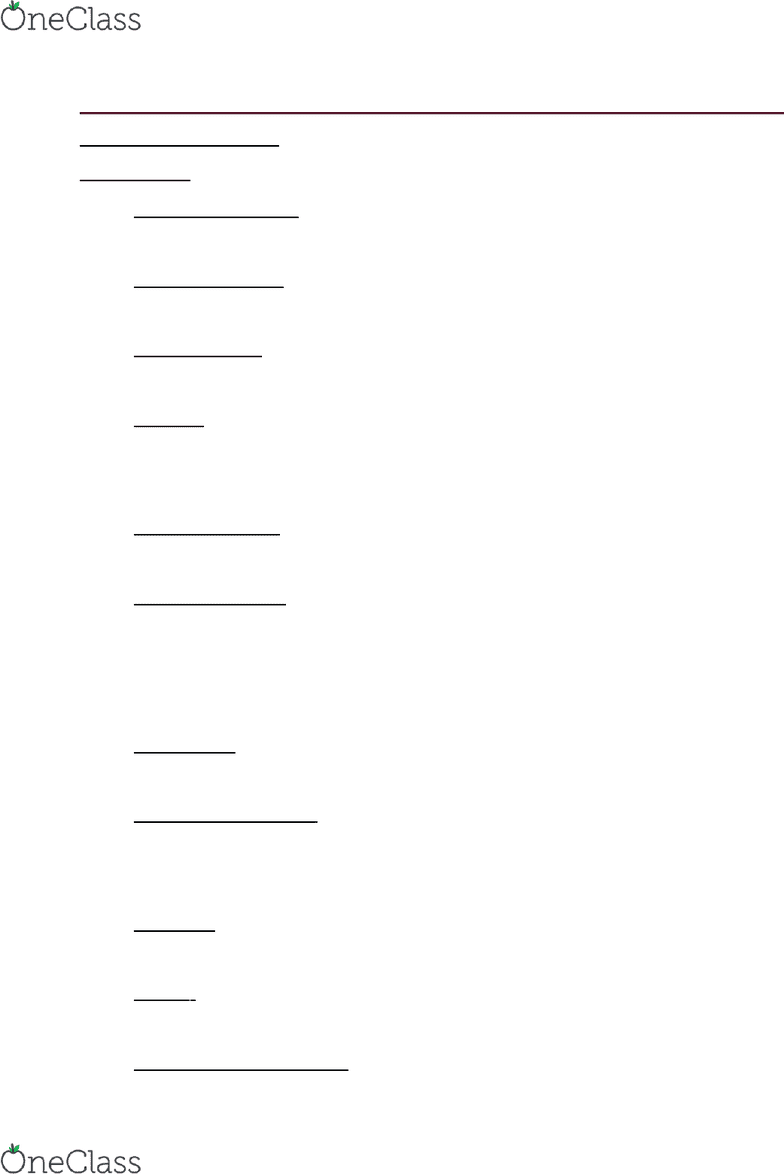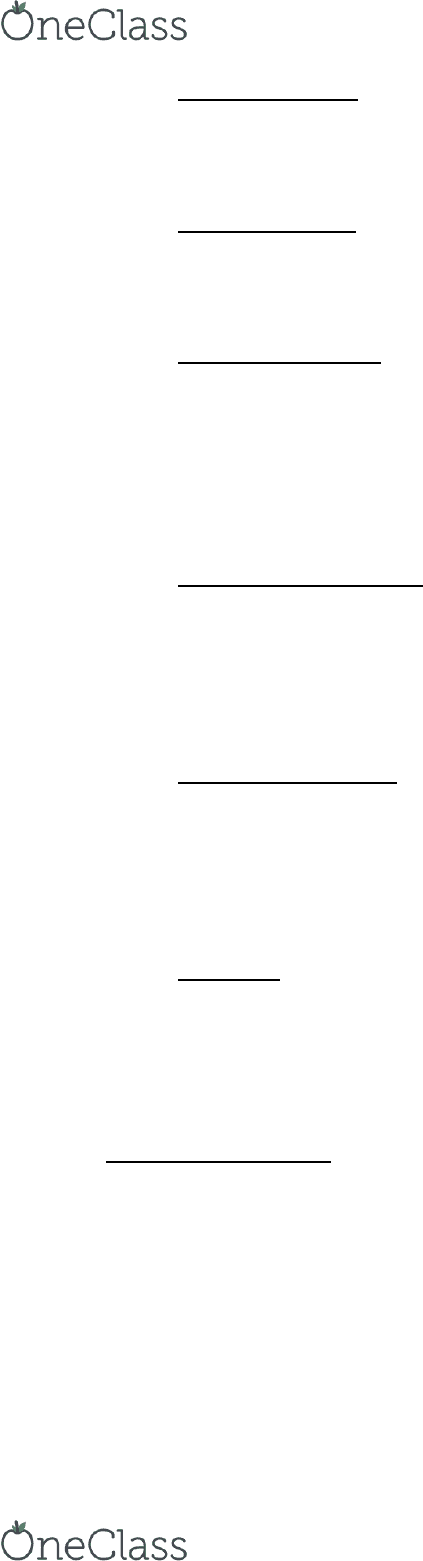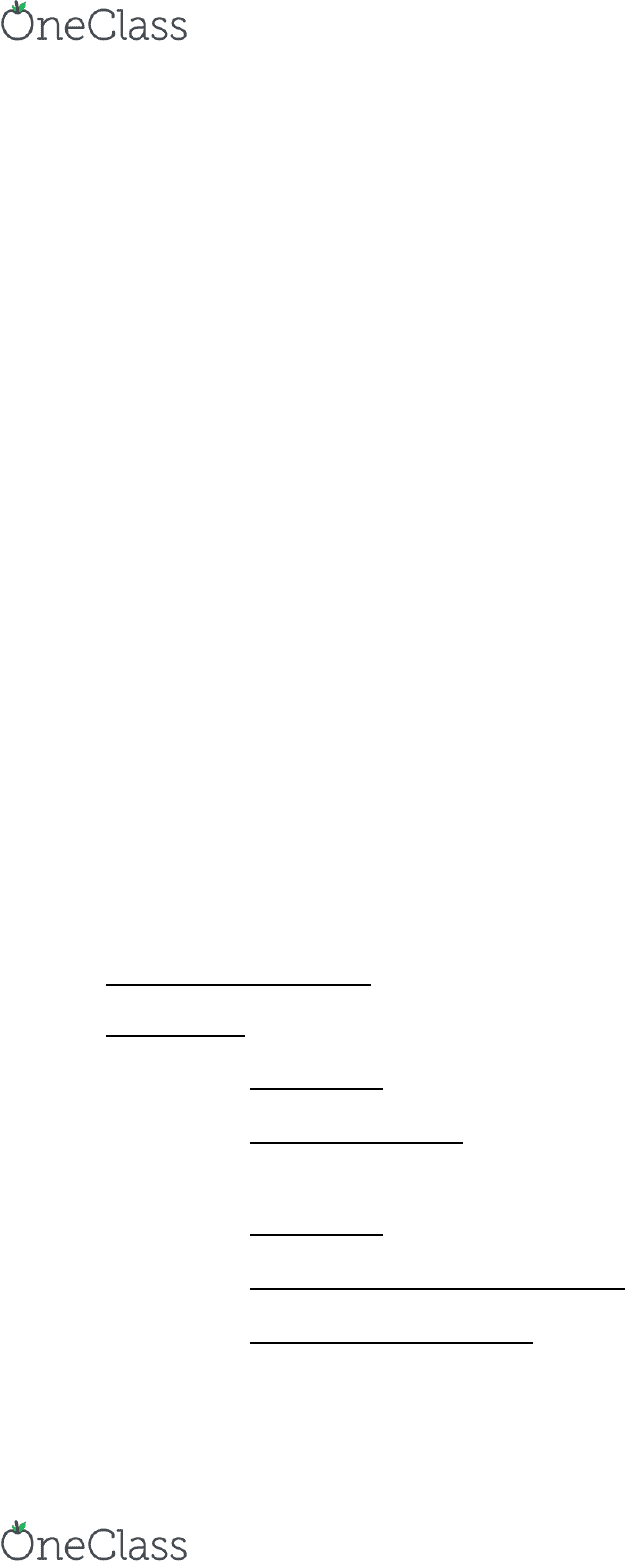Study Guides (390,000)
CA (150,000)
UBC (5,000)
KIN (70)
Midterm

# KIN 151 Study Guide - Midterm Guide: Free Body Diagram, Mechanical Advantage, Simple Machine

Department
Kinesiology
Course Code
KIN 151
Professor
Paul Kennedy
Study Guide
Midterm

This preview shows pages 1-3. to view the full 11 pages of the document.1. 2nd Section Study Guides – KIN 151
Class 11 Study Guide
Definitions:
Center of gravity: The point around which a bodys weight is equally
balanced, no matter how body is positioned
Center of Mass: The point around which the mass and weight of a
body are balanced, no matter how body is positioned
Centric Force: Acts on on the center of a system, produce translation
(linear motion only)
Couple: Pair of equal, oppositely directed forces that act on opposite
sides of an axis of rotation to produce torque
otwo eccentric forces that cause rotation ONLY
Eccentric Force: act off the center of a system, produce translation
and rotation (rotation and curvilinear motion)
First Class Lever: lever positioned with the applied force and resistance
on opposite sides of the axis of rotation
oSee saw example
oMechanical advantage may be greater or less than 1
Force Arm: shortest perpendicular distance between a forces line of
action and axis of rotation
Free body diagram : a graphical illustration used to visualize the
applied forces and resulting reactions on a body
oProblem solving strategy, not meant to be scaled
Fulcrum: The point of support, or axis, about which a lever may be
Lever: A simple machine consisting of a relatively rigid, barlike body
Mechanical Advantage: ratio of force arm to resistance for a given
lever
find more resources at oneclass.com
find more resources at oneclass.com

Only pages 1-3 are available for preview. Some parts have been intentionally blurred.Moment Arm: Also referred to as force arm, the perpendicular
distance between the line of action of the force and the axis of
rotation
Pure Moment: When two forces that are equal in magnitude but
opposite in direction, act equidistant (at equal distances) from the
center of gravity, result = pure moment
Resistance Arm: The perpendicular distance from the forces line of
action to the axis of rotation (the output force)
oThe moment arm of an applied force can also be referred to as
the force arm, and the moment arm of a RESISTANCE can be
referred to as the resistance arm
Second Class Lever: Lever positioned with the resistance between the
applied force and the fulcrum
oWheel barrow example
oMechanical advantage always greater than 1
Third Class Lever: Lever positioned with the applied force between the
fulcrum and the resistance
oNut cracker example
oMechanical advantage always less than 1
Torque: A force that produces rotation
oThe rotary effect of a force about an axis of rotation, measured
as the product of the force and the perpendicular distance
between the forces line of action and the axis
Sample Questions
1. What type of force produces both translation and rotation?
a. Eccentric forces
2. Identify the factors that influence the size of torque?
a. The magnitude of the force
find more resources at oneclass.com
find more resources at oneclass.com

Only pages 1-3 are available for preview. Some parts have been intentionally blurred.a.i. The larger the applied force the bigger the rotational
change
b. Point of application
b.i. The distance between the force and the axis of rotation
c. Direction
c.i. The perpendicular distance from the force arm to the axis
of rotation
3. Define the term mechanical advantage? What does the mechanical
a. Mechanical Advantage = ratio of force arm to resistance arm for
a given lever
a.i. Tells us mechanical effectiveness of a lever
a.ii. Mechanical advantage increases the lever is built for
strength but sacrifices speed
4. A wheel barrow is an example of which type of lever? What type of
advantage do second class levers offer?
a. Wheelbarrow = second class lever
a.i. Resistance is in middle, this magnifies muscle and makes it
as strong as possible
Class 12 Study Guide
Definitions
Abduction
Absolute Angle – The space between a body segment with
respect to a fixed line of reference